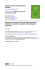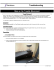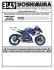# THREE DIMENSIONAL SYSTEMS Lecture 6: The Lorenz Equations

## Transcription

THREE DIMENSIONAL SYSTEMS Lecture 6: The Lorenz Equations
```THREE DIMENSIONAL
SYSTEMS
Lecture 6: The Lorenz
Equations
6. The Lorenz (1963) Equations
The Lorenz equations were originally derived
by Saltzman (1962) as a ‘minimalist’ model
of thermal convection in a box
ẋ = σ(y − x)
(1)
ẏ = rx − y − xz
(2)
ż = xy − bz
(3)
where σ (“Prandtl number”), r (“Rayleigh
number”) and b are parameters (> 0). These
equations also arise in studies of convection
and instability in planetary atmospheres, models of lasers and dynamos etc. Willem Malkus
also devised a water-wheel demonstration...
1
Malkus’ water wheel
This simple-looking deterministic system
turns out to have “interesting” erratic
dynamics!
2
8.1 Simple properties of the Lorenz Equations
• Nonlinearity - the two nonlinearities are
xy and xz
• Symmetry - Equations are invariant under (x, y) → (−x, −y). Hence if (x(t), y(t), z(t))
is a solution, so is (−x(t), −y(t), z(t))
• Volume contraction - The Lorenz system
is dissipative i.e. volumes in phase-space
contract under the flow
• Fixed points - (x∗, y ∗, z ∗) = (0, 0, 0) is a
fixed point for all values of the parameters. For r > 1 there is alsoqa pair of
fixed points C ± at x∗ = y ∗ = ± b(r − 1),
z ∗ = r −1. These coalesce with the origin
as r → 1+ in a pitchfork bifurcation
3
Linear stability of the origin
Linearization of the original equations about
the origin yields
ẋ = σ(y − x)
ẏ = rx − y
ż = −bz
Hence, the z-motion decouples, leaving
ẋ
ẏ
!
=
−σ σ
r −1
!
x
y
!
with trace τ = −σ − 1 < 0 and determinant
∆ = σ(1 − r).
For r > 1, origin is a saddle point since ∆ < 0
(see Lecture 4)
For r < 1, origin is a sink since τ 2 − 4∆ =
(σ + 1)2 − 4σ(1 − r) = (σ − 1)2 + 4στ > 0 →
a stable node.
Actually for r < 1 it can be shown that every
trajectory approaches the origin as t → ∞ the
origin is globally stable, hence there can be
no limit cycles or chaos for r < 1.
4
Subcritical Hopf bifurcation occurs at
σ(σ + b + 3)
r=
≡ rH > 1
σ−b−1
assuming that σ − b − 1 > 0.
Fig. 6.1.1 - (Partial bifurcation diagram)
Recall from Lecture 5 that at a subcritical
Hopf bifurcation trajectories must fly off to
a distant attractor. This 2nd attractor must
have some strange properties, since any limit
cycles for r > rH are unstable (cf “proof”
by Lorenz). The trajectories for r > rH are
therefore continually being repelled from one
unstable object to another. At the same
time, they are confined to a bounded set of
zero volume, yet manage to move in this set
forever without intersecting - a strange attractor!
5
6.2 Chaos on a Strange Attractor
Lorenz considered the case σ = 10, b = 8/3, r =
28 with (x0, y0, z0) = (0, 1, 0).
NB rH = σ(σ + b + 3)/(σ − b − 1) ' 24.74,
hence r > rH . The resulting solution y(t)
looks like...
Fig. 6.2.1
After an initial transient, the solution settles
into an irregular oscillation that persists as
t → ∞ but never repeats exactly. The motion
is aperiodic.
6
Lorenz discovered that a wonderful structure
emerges if the solution is visualized as a trajectory in phase space. For instance, when
x(t) is plotted against z(t), the famous butterfly wing pattern appears
Fig. 6.2.2
• The trajectory appears to cross itself repeatedly, but that’s just an artifact of
projecting the 3-dimensional trajectory onto
a 2-dimensional plane. In 3-D no crossings occur!
7
• The number of circuits made on either
side varies unpredictably from one cycle
to the next. The sequence of the number
of circuits in each lobe has many of the
characteristics of a random sequence!
• When the trajectory is viewed in all 3
dimensions, it appears to settle onto a
thin set that looks like a pair of butterfly
wings. We call this attractor a strange
attractor and it can be shown schematically as...
Fig. 6.2.3
8
What is the geometric structure of the strange
attractor?
The uniqueness theorem means that trajectories cannot cross or merge, hence the two
surfaces of the strange attractor can only appear to merge.
Lorenz concluded that “there is an infinite
complex of surfaces” where they appear to
merge. Today this “infinite complex of surfaces” would be called a FRACTAL.
A fractal is a set of points with zero volume
but infinite surface area.
Fractals will be discussed later after a closer
look at chaos...
9
Exponential divergence of nearby trajectories
The motion on the attractor exhibits sensitive dependence on initial conditions. Two
trajectories starting very close together will
rapidly diverge from each other, and thereafter have totally different futures. The practical implication is that long-term prediction
becomes impossible in a system like this, where
small uncertainties are amplified enormously
fast.
Suppose we let transients decay so that the
trajectory is “on” the attractor. Suppose
x(t) is a point on the attractor at time t,
and consider a nearby point, say x(t) + δ(t),
where δ is a tiny separation vector of initial
length ||δ0|| = 10−15 , say
Fig. 6.2.4
10
Sensitivity to initial conditions on Lorenz attractor
11
In numerical studies of the Lorenz attractor,
one finds that ||δ(t)|| ∼ ||δ0||eλt, where λ '
0.9.
Hence neighbouring trajectories separate exponentially fast!
Equivalently, if we plot ln ||δ(t)|| versus t, we
find a curve that is close to a straight line
with a positive slope λ.
Fig. 6.2.5
12
Note...
• The curve is never straight, but has wiggles since the strength of exponential divergence varies somewhat along the attractor.
• The exponential divergence must stop when
the separation is comparable to the “diameter” of the attractor - the trajectories cannot get any further apart! (curve
saturates for large t)
• The number λ is often called the Lyapunov exponent, though this is somewhat
sloppy terminology....
13
This is because...
(i) There are actually n different Lyapunov
exponents for an n-dimensional system, defined as follows...
Consider the evolution of an infinitesimal sphere
(in phase space) of perturbed initial conditions. During its evolution the sphere becomes distorted into an infinitesimal ellipsoid.
Let δk (t), k = 1, 2, 3...n denote the length of
the kth principal axis of the ellipsoid. Then
δk (t) ∼ δk (0)eλk t,
where the λk are the Lyapunov exponents.
For large t, the diameter of the ellipsoid is
controlled by the most positive λk . Thus our
λ above is actually the largest Lyapunov exponent.
(ii) λ depends (slightly) on which trajectory
we study. We should really average over
many different points on the same trajectory
to get the true value of λ.
14
When a system has a positive Lyapunov exponent, there is a time horizon beyond which
prediction will break down....
Fig. 6.2.6
Suppose we measure the initial conditions of
an experimental system very accurately. Of
course no measurement is perfect - there is
always some error ||δ0|| between our estimate
and the true initial state. After a time t the
discrepancy grows to ||δ(t)|| ∼ ||δ0||eλt.
15
Let a be a measure of our tolerance, i.e. if
a prediction is within a of the true state, we
consider it acceptable. Then our prediction
becomes intolerable when ||δ(t)|| ≥ a, i.e. after a time
thorizon ∼ O
a
1
ln
λ ||δ||
!
The logarithmic dependence on ||δ0|| is what
hurts....!
Example Suppose a = 10−3, ||δ0|| = 10−7.
⇒ thorizon =
4 ln 10
λ
If we improve the initial error to ||δ0|| = 10−13 ,
10 ln 10
⇒ thorizon =
λ
i.e. only 10/4 = 2.5 times longer!
16
Defining Chaos
No definition of the term “chaos” is universally accepted - even now! - but almost everyone would agree on the three ingredients
used in the following working definition:
Chaos is aperiodic long-term behaviour in
a deterministic system that exhibits sensitive dependence on initial conditions
1. Aperiodic long-term behaviour means that
there are trajectories which do not settle
down to fixed points, periodic or quasiperiodic orbits as t → ∞.
2. Deterministic means that the system has
no random or noisy inputs or parameters.
Irregular behaviour arises solely from the
system’s nonlinearity.
17
3. Sensitive dependence on initial conditions
means that nearby trajectories diverge exponentially fast, i.e. the system has at
least one positive Lyapunov exponent.
Some people think that chaos is just a fancy
word for instability. For example, the system
ẋ = x is deterministic and shows exponential separation of nearby trajectories. However, we should not consider this system to
be chaotic!
Trajectories are repelled to infinity, and never
return. Hence infinity is a fixed point of the
system, and ingredient 1. above specifically
excludes fixed points!
18
Defining “attractor” and “strange attractor”
The term attractor is also difficult to define
in a rigorous way. Loosely, an attractor is a
set of points to which all neighbouring trajectories converge. Stable fixed points and
stable limit cycles are examples.
More precisely, we define an attractor to be
a closed set A with the following properties:
1. A is an invariant set: any trajectory x(t)
that starts in A stays in A for all time.
2. A attracts an open set of initial conditions: there is an open set U containing
A such that if x(0) ∈ U , then the distance from x(t) to A tends to zero as
t → ∞. Hence A attracts all trajectories
that start sufficiently close to it. The
largest such U is called the basin of attraction of A.
19
3. A is minimal: there is no proper subset
of A that satisfies conditions 1. and 2.
Example
(
ẋ = µx − x3
ẏ =
−y
Let I denote the interval −1 ≤ x ≤ 1, y = 0.
Is I an attractor?
Fig. 6.2.7
So, I is an invariant set (condition 1.). Also,
I attracts an open set of initial conditions
- it attracts all trajectories in the xy-plane.
But I is not an attractor because it is not
minimal. The stable fixed points (±1, 0) are
proper subsets of I that also satisfy conditions 1. and 2. These points are the only
attractors for the system.
20
Maybe the same is true for the Lorenz Equations? - Nobody has yet proved that the
Lorenz attractor is truly an attractor!!
Finally we define a strange attractor to be an
attractor that exhibits sensitive dependence
on initial conditions.
Strange attractors were originally called strange
because they are often (but not always...)
fractal sets. Nowadays, this geometric property is regarded as less important than the
dynamical property of sensitive dependence
on initial conditions. The terms “chaotic
attractor” and “fractal attractor” are used
when one wishes to emphasize one or other
of these aspects.
21
The Lorenz Map
Consider the following view of the Lorenz
strange attractor...
Fig. 6.3.1
Lorenz wrote that “the trajectory apparently
leaves one spiral only after exceeding some
critical distance from the centre... It therefore seems that some single feature of a given
circuit should predict the same feature of the
following circuit.”
22
The “single feature” he focused on was zn,
the nth local maximum of z(t).
Fig. 6.3.2
Lorenz’s idea is that zn should predict zn+1.
He checked this by numerical integration. The
plot of zn+1 vs zn looks like....
Fig. 6.3.3
23
The data from the chaotic time series appears to fall neatly on a curve - there is no
“thickness” to the graph. Hence Lorenz was
able to extract order from chaos!
The function zn+1 = f (zn ) is now called the
Lorenz Map. Note...
• The graph is not actually a curve - it does
have some thickness so, strictly speaking,
f (z) is not a well-defined function.
• The Lorenz Map reminds us of the Poincaré
map, but there is a distinction - the Poincaré
map tells us how the two coordinates of
a point on a surface change after first
map characterises the trajectory by only
one number. This approach only works
if the attractor is very “flat” (close to
2-D).
24
Exploring Parameter Space
So far we have concentrated mainly on the
case σ = 10, b = 8/3, r = 28 as in Lorenz’s
original work published in 1963.
Changing parameters is like a walk through
the jungle! One finds many sorts of exotic
behaviours, such as exotic limit cycles tied
in knots, pairs of limit cycles linked through
each other, intermittent chaos, noisy periodicity.....as well as strange attractors! In fact
much remains to be discovered....
Fig. 6.4.1
25
Example Consider r = 21, with σ = 10 and
b = 8/3 as usual. The solution exhibits transient chaos, in which the trajectory seems to
be tracing a strange attractor, but eventually
spirals towards C + or C −...
Fig. 6.4.2
Fig. 6.4.3
26
The long-term behaviour is not aperiodic hence the dynamics are not chaotic. However, the dynamics do exhibit sensitive dependence on initial conditions - the trajectory
could end up on either C + or C − - hence the
system’s behaviour is unpredictable.
Transient chaos shows that a deterministic
system can be unpredictable, even if its final states are very simple. This is familiar from everyday experience - many games
of “chance” used in gambling are essentially
demonstrations of transient chaos....e.g. a
rolling dice...
You don’t need strange attractors to generate effectively random behaviour!
27
It turns out that for large r the dynamics become simple again - the system has a global
attracting limit cycle for r > 313.
Example Consider r = 350, with σ = 10 and
b = 8/3 as usual.
Fig. 6.4.4
Fig. 6.4.5
28
```

### Lorenz system II - Harvard Mathematics Department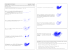r < 24.06, these cycles come closer to the fixed points C ± . The picture shows the parameter r = 20.

### dr. edward norton loreNZ - University of Wisconsin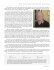### Vadim S. Anishchenko. - Dynamics, Bifurcations, and Strange### Uses of persistence for interpreting coarse instructions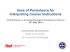### 054-083 MNB MA11.indd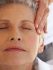### Effective Fokker-Planck equation for birhythmic modified van der Pol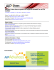### PrimeFilm 1800 AFL Low-Cost 35mm Slide/Film Scanning Through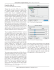### THE BUTTERFLY EFFECT### Extended phase diagram of the Lorenz model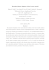### t - Open Access Home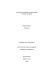### Extreme value laws in dynamical systems under physical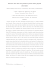### R - imeko### this product is designed for use in closed course racing### Intelligence in Living Cells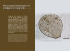### Lapidus Bunionectomy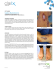### Bifurcations of periodic and chaotic attractors in pinball### Snakeboard Motion Planning with Viscous Friction and Skidding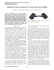### ARTICLE IN PRESS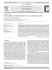### Dynamical systems with generalized hyperbolic attractors